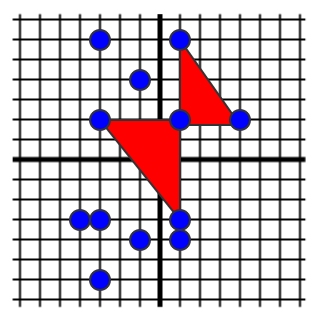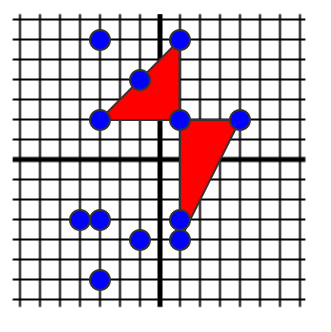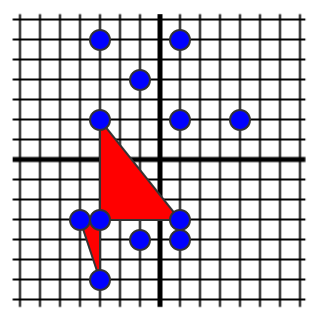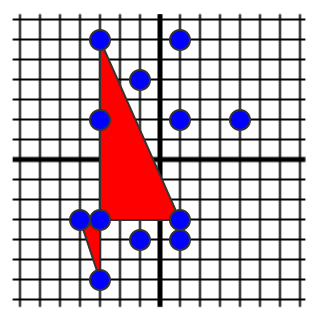2016-CCOQR-Q1 Stupendous Bowties

# 2016-CCOQR-Q1 Stupendous Bowties

Problem Description
View Problem

## Key Insight #1

The problem description for this problem can be confusing at first. To help you understand what constitutes a studpendous bowtie and what does not we have shown below the eight possible bowties formed from the sample input.## Key Insight #2

We can see from the above diagrams that each bowtie is mirrored. This means that for one set of five points that form a bowtie, form two. If we pick a point to use as the spectacular vertex. The number of bowties possible is equal to the number of points on the left * points on the right * point above * points below * 2. We only care about points on the same x value or y value as the spectacular vertex. We can see that this is a searching algorithm.

Brute Force Implementation

A brute force solution that receives 7/15 points and uses two multimaps to store and retreive the points.

View Brute Force Solution

## Optimized Searching Algorithm

Our optimized searching algorithm involves sorting the points based on their x values and then again based on their y values. To do this efficiently we will use an index sort. We will keep the points in one static array and we will create two arrays for the index sort, one for x and one for y. We will iterate through the x sorted array looking for blocks of points with the same x value. If we find a block that is at least three points, then we evaluate the number of bowties formed by each of the points excluding the first and last. To do this we will look for points with the same y value by looking in the y sorted array. To move from the x sorted array to the y sorted array effeciently, without searching through the entire y sorted array, we will use an inverted index sorted array to speed up this process. Below is a table illustrating this method using the sample data set.

Index Points X Sorted Index Y Sorted Index Y Sorted Inverted Index
0 -3, 6 6 (-4, -3) 11 (-3, -6) 10
1 1, 6 11 (-3, -6) 9 (-1, -4) 11
2 -1, 4 7 (-3, -3) 10 (1, -4) 9
3 -3, 2 3 (-3, 2) 6 (-4, -3) 6
4 1, 2 0 (-3, 6) 7 (-3, -3) 7
5 4, 3 9 (-1, -4) 8 (1, -3) 8
6 -4, -3 2 (-1, 4) 3 (-3, 2) 3
7 -3, -3 10 (1, -4) 4 (1, 2) 4
8 1, -3 8 (1, -3) 5 (4, 2) 5
9 -1, -4 4 (1, 2) 2 (-1, 4) 1
10 1, -4 1 (1, 6) 0 (-3, 6) 2
11 -3, -6 5 (4, 2) 1 (1, 6) 0

As you can see the X Sorted Index array gives us the index of the point not the actual points (the points are shown in parenthesis). The indexes are what is actually sorted. If we walk through the X Sorted array the first block that we find is points with an X value of negative three.

Index Points X Sorted Index Y Sorted Index Y Sorted Inverted Index
0 -3, 6 6 (-4, -3) 11 (-3, -6) 10
1 1, 6 11 (-3, -6) 9 (-1, -4) 11
2 -1, 4 7 (-3, -3) 10 (1, -4) 9
3 -3, 2 3 (-3, 2) 6 (-4, -3) 6
4 1, 2 0 (-3, 6) 7 (-3, -3) 7
5 4, 3 9 (-1, -4) 8 (1, -3) 8
6 -4, -3 2 (-1, 4) 3 (-3, 2) 3
7 -3, -3 10 (1, -4) 4 (1, 2) 4
8 1, -3 8 (1, -3) 5 (4, 2) 5
9 -1, -4 4 (1, 2) 2 (-1, 4) 1
10 1, -4 1 (1, 6) 0 (-3, 6) 2
11 -3, -6 5 (4, 2) 1 (1, 6) 0

There are four points in this block. We ignore the first and last points (yellow background) because there is not points above the first and no points below the last. The second and third (pink background)are the interesting ones. They could potentially form bowties. We start with the second in the block, point 7 (-3, -3), we know that there is one point above and two below. We need to find how many are left and how many are right. We need to switch to the Y Sorted array. We can use the inverted array to do this. We are looking at point 7 so we look at index 7 in the inverted array.

Index Points X Sorted Index Y Sorted Index Y Sorted Inverted Index
0 -3, 6 6 (-4, -3) 11 (-3, -6) 10
1 1, 6 11 (-3, -6) 9 (-1, -4) 11
2 -1, 4 7 (-3, -3) 10 (1, -4) 9
3 -3, 2 3 (-3, 2) 6 (-4, -3) 6
4 1, 2 0 (-3, 6) 7 (-3, -3) 7
5 4, 3 9 (-1, -4) 8 (1, -3) 8
6 -4, -3 2 (-1, 4) 3 (-3, 2) 3
7 -3, -3 10 (1, -4) 4 (1, 2) 4
8 1, -3 8 (1, -3) 5 (4, 2) 5
9 -1, -4 4 (1, 2) 2 (-1, 4) 1
10 1, -4 1 (1, 6) 0 (-3, 6) 2
11 -3, -6 5 (4, 2) 1 (1, 6) 0

The number there is 4 so we look in the Y Sorted array at index 4 and we find point 7 (the same point - both are pink background). We can then look around to see how many points are left and how many points are right. We then multiply the left by the right by the points above and by the points below.

Index Points X Sorted Index Y Sorted Index Y Sorted Inverted Index
0 -3, 6 6 (-4, -3) 11 (-3, -6) 10
1 1, 6 11 (-3, -6) 9 (-1, -4) 11
2 -1, 4 7 (-3, -3) 10 (1, -4) 9
3 -3, 2 3 (-3, 2) 6 (-4, -3) 6
4 1, 2 0 (-3, 6) 7 (-3, -3) 7
5 4, 3 9 (-1, -4) 8 (1, -3) 8
6 -4, -3 2 (-1, 4) 3 (-3, 2) 3
7 -3, -3 10 (1, -4) 4 (1, 2) 4
8 1, -3 8 (1, -3) 5 (4, 2) 5
9 -1, -4 4 (1, 2) 2 (-1, 4) 1
10 1, -4 1 (1, 6) 0 (-3, 6) 2
11 -3, -6 5 (4, 2) 1 (1, 6) 0

We need to find how many are left and how many are right. We need to switch to the Y Sorted array. We can use the inverted array to do this. We are looking at point 7 so we look at index 7 in the inverted array and we find one point left and right - yellow background),.

This number is finally multiplied by 2.

## Further Optimization

To optimize the process of searching for points left and right further, we can create two more arrays called Y Sorted Left and Y Sorted Right and we iterate through the Y Sorted array from start to finish and from finish to start to generate these arrays. The original Y Sorted Index was (Y point values that determine sorting order in red):

Y Sorted Index Vale (X,Y)
0 11 (-3,-6)
1 9 (-1,-4)
2 10 (1,-4)
3 6 (-4,-3)
4 7 (-3, -3)
5 8 (1,-3)
6 3 (-3,2)
7 4 (1,2)
8 5 (4,2)
9 2 (-1,4)
10 0 (-3,6)
11 1 (1,6)

We create a left sorted array by counting the number of values left of the current value that have the same Y:

Y Sorted Index Vale (X,Y) Y Sorted Left
0 11 (-3,-6) 0
1 9 (-1,-4) 0
2 10 (1,-4) 1
3 6 (-4,-3) 0
4 7 (-3, -3) 1
5 8 (1,-3) 2
6 3 (-3,2) 0
7 4 (1,2) 1
8 5 (4,2) 2
9 2 (-1,4) 0
10 0 (-3,6) 0
11 1 (1,6) 1

The right sorted array is created in the same way:

Y Sorted Index Vale (X,Y) Y Sorted Right
0 11 (-3,-6) 0
1 9 (-1,-4) 1
2 10 (1,-4) 0
3 6 (-4,-3) 2
4 7 (-3, -3) 1
5 8 (1,-3) 0
6 3 (-3,2) 2
7 4 (1,2) 1
8 5 (4,2) 0
9 2 (-1,4) 0
10 0 (-3,6) 1
11 1 (1,6) 0

These two arrays allow the search for how many points have the same Y value left and right to require a lookup on each array.

Please attempt to solve the problem on your own before viewing the solution!
View Solution